The SURVEYREG Procedure

Displayed Output

Subsections:

The SURVEYREG procedure produces output that is described in the following sections.

Output that is generated by the EFFECT, ESTIMATE, LSMEANS, LSMESTIMATE, and SLICE statements is not listed below. For information about the output that is generated by these statements, see the corresponding sections of Chapter 19: Shared Concepts and Topics.

Data Summary

By default, PROC SURVEYREG displays the following information in the "Data Summary" table:

• Number of Observations, which is the total number of observations used in the analysis, excluding observations with missing values

• Sum of Weights, if you specify a WEIGHT statement

• Mean of the dependent variable in the MODEL statement, or Weighted Mean if you specify a WEIGHT statement

• Sum of the dependent variable in the MODEL statement, or Weighted Sum if you specify a WEIGHT statement

Design Summary

When you specify a CLUSTER statement or a STRATA statement, the procedure displays a "Design Summary" table, which provides the following sample design information:

• Number of Strata, if you specify a STRATA statement

• Number of Strata Collapsed, if the procedure collapses strata

• Number of Clusters, if you specify a CLUSTER statement

• Overall Sampling Rate used to calculate the design effect, if you specify the DEFF option in the MODEL statement

Domain Summary

By default, PROC SURVEYREG displays the following information in the "Domain Summary" table:

• Number of Observations, which is the total number of observations used in the analysis

• total number of observations in the current domain

• total number of observations not in the current domain

• Sum of Weights for the observations in the current domain, if you specify a WEIGHT statement

Fit Statistics

By default, PROC SURVEYREG displays the following regression statistics in the "Fit Statistics" table:

• R-square for the regression

• Root MSE, which is the square root of the mean square error

• Denominator DF, which is the denominator degrees of freedom for the F tests and also the degrees of freedom for the t tests produced by the procedure

Variance Estimation

If the variance method is not Taylor series (see the section Variance Estimation) or if the NOMCAR option is used, by default, PROC SURVEYREG displays the following variance estimation information in the "Variance Estimation" table:

• Method, which is the variance estimation method

• Number of Replicates, if you specify the VARMETHOD=BRR or VARMETHOD=JACKKNIFE option

• Fay Coefficient, if you specify the VARMETHOD=BRR(FAY) method-option

• Replicate Weights input data set name, if you provide replicate weights with a REPWEIGHTS statement

• Missing Levels, which indicates whether missing levels of categorical variables are included by the MISSING option

• Missing Values, which indicates whether observations with missing values are included in the analysis by the NOMCAR option

Stratum Information

When you specify the LIST option in the STRATA statement, PROC SURVEYREG displays a "Stratum Information" table, which provides the following information for each stratum:

• Stratum Index, which is a sequential stratum identification number

• STRATA variable(s), which lists the levels of STRATA variables for the stratum

• Population Total, if you specify the TOTAL= option

• Sampling Rate, if you specify the TOTAL= option or the RATE= option. If you specify the TOTAL= option, the sampling rate is based on the number of nonmissing observations in the stratum.

• N Obs, which is the number of observations

• number of Clusters, if you specify a CLUSTER statement

• Collapsed, which has the value 'Yes' if the stratum is collapsed with another stratum before analysis

If PROC SURVEYREG collapses strata, the "Stratum Information" table also displays stratum information for the new, collapsed stratum. The new stratum has a Stratum Index of 0 and is labeled 'Pooled.'

Class Level Information

If you use a CLASS statement to name classification variables, PROC SURVEYREG displays a "Class Level Information" table. This table contains the following information for each classification variable:

• CLASS Variable, which lists each CLASS variable name

• Levels, which is the number of values or levels of the classification variable

• Values, which lists the values of the classification variable. The values are separated by a white space character; therefore, to avoid confusion, you should not include a white space character within a classification variable value.

X’X Matrix

If you specify the XPX option in the MODEL statement, PROC SURVEYREG displays thematrix. When there is a WEIGHT variable, the procedure displays the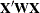matrix. This option also displays the crossproducts vector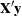or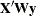, whereis the response vector (dependent variable).

Inverse Matrix of X’X

If you specify the INVERSE option in the MODEL statement, PROC SURVEYREG displays the inverse or the generalized inverse of thematrix. When there is a WEIGHT variable, the procedure displays the inverse or the generalized inverse of thematrix.

ANOVA for Dependent Variable

If you specify the ANOVA option in the model statement, PROC SURVEYREG displays an analysis of variance table for the dependent variable. This table is identical to the ANOVA table displayed by the GLM procedure.

Tests of Model Effects

By default, PROC SURVEYREG displays a "Tests of Model Effects" table, which provides Wald’s F test for each effect in the model. The table contains the following information for each effect:

• Effect, which is the effect name

• Num DF, which is the numerator degrees of freedom for Wald’s F test

• F Value, which is Wald’s F statistic

• Pr > F, which is the significance probability corresponding to the F Value

A footnote displays the denominator degrees of freedom, which is the same for all effects.

Estimated Regression Coefficients

PROC SURVEYREG displays the "Estimated Regression Coefficients" table by default when there is no CLASS statement. Also, the procedure displays this table when you specify a CLASS statement and also specify the SOLUTION option in the MODEL statement. This table contains the following information for each regression parameter:

• Parameter, which identifies the effect or regressor variable

• Estimate, which is the estimate of the regression coefficient

• Standardized Estimate, which is the standardized regression coefficient

• Standard Error, which is the standard error of the estimate

• t Value, which is the t statistic for testing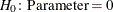• Pr > | t |, which is the two-sided significance probability corresponding to the t Value

Covariance of Estimated Regression Coefficients

When you specify the COVB option in the MODEL statement, PROC SURVEYREG displays the "Covariance of Estimated Regression Coefficients" matrix.

Coefficients of Contrast

When you specify the E option in a CONTRAST statement, PROC SURVEYREG displays a "Coefficients of Contrast" table for the contrast. You can use this table to check the coefficients you specified in the CONTRAST statement. Also, this table gives a note for a nonestimable contrast.

Analysis of Contrasts

If you specify a CONTRAST statement, PROC SURVEYREG produces an "Analysis of Contrasts" table, which displays Wald’s F test for the contrast. If you use more than one CONTRAST statement, the procedure displays all results in the same table. The "Analysis of Contrasts" table contains the following information for each contrast:

• Contrast, which is the label of the contrast

• Num DF, which is the numerator degrees of freedom for Wald’s F test

• F Value, which is Wald’s F statistic for testing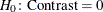• Pr > F, which is the significance probability corresponding to the F Value